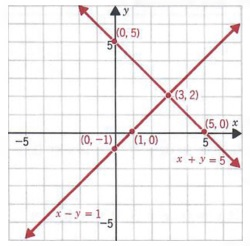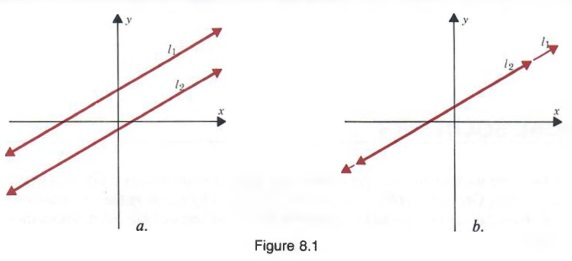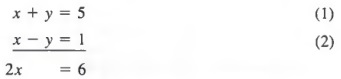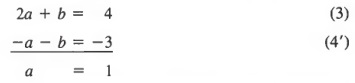Algebra

Equations

Inequalities

Graphs

Numbers

Calculus

Matrices

Tutorials

Enter an equation or system of equations, enter the variable or variables to be solved for, set the options and click the Solve button.

Equation(s)

[one per line]
Variable(s)

[one per line]
Options
 Approximate to
digits

 Eliminate variables

[one per line]

# SYSTEMS OF LINEAR EQUATIONS

## GRAPHICAL SOLUTIONS

Often, we want to find a single ordered pair that is a solution to two different linear equations. One way to obtain such an ordered pair is by graphing the two equations on the same set of axes and determining the coordinates of the point where they intersect.

Example 1

Graph the equations

x + y = 5

x - y = 1

on the same set of axes and determine the ordered pair that is a solution for each equation.

Solution

Using the intercept method of graphing, we find that two ordered pairs that are solutions of x + y = 5 are

(0, 5) and (5, 0)

And two ordered pairs that are solutions of

x - y = 1 are

(0,-1) and (1,0)

The graphs of the equations are shown.

The point of intersection is (3, 2). Thus, (3, 2) should satisfy each equation.

In fact, 3 + 2 = 5 and 3 - 2 = 1In general, graphical solutions are only approximate. We will develop methods for exact solutions in later sections.

Linear equations considered together in this fashion are said to form a system of equations. As in the above example, the solution of a system of linear equations can be a single ordered pair. The components of this ordered pair satisfy each of the two equations.

Some systems have no solutions, while others have an infinite number of solu- tions. If the graphs of the equations in a system do not intersect-that is, if the lines are parallel (see Figure 8.1a)-the equations are said to be inconsistent, and there is no ordered pair that will satisfy both equations. If the graphs of the equations are the same line (see Figure 8.1b), the equations are said to be dependent, and each ordered pair which satisfies one equation will satisfy both equations. Notice that when a system is inconsistent, the slopes of the lines are the same but the y-intercepts are different. When a system is dependent, the slopes and y-intercepts are the same.In our work we will be primarily interested in systems that have one and only one solution and that are said to be consistent and independent. The graph of such a system is shown in the solution of Example 1.

## SOLVING SYSTEMS BY ADDITION I

We can solve systems of equations algebraically. What is more, the solutions we obtain by algebraic methods are exact.

The system in the following example is the system we considered in Section 8.1 on page 335.

Example 1

Solve

x + y = 5 (1)

x - y = 1 (2)

Solution
We can obtain an equation in one variable by adding Equations (1) and (2)Solving the resulting equation for x yields

2x = 6, x = 3

We can now substitute 3 for x in either Equation (1) or Equation (2) to obtain the corresponding value of y. In this case, we have selected Equation (1) and obtain

(3) + y = 5

y = 2

Thus, the solution is x = 3, y = 2; or (3, 2).

Notice that we are simply applying the addition property of equality so we can obtain an equation containing a single variable. The equation in one variable, together with either of the original equations, then forms an equivalent system whose solution is easily obtained.

In the above example, we were able to obtain an equation in one variable by adding Equations (1) and (2) because the terms +y and -y are the negatives of each other. Sometimes, it is necessary to multiply each member of one of the equations by -1 so that terms in the same variable will have opposite signs.

Example 2

Solve

2a + b = 4 (3)

a + b = 3 (4)

Solution

We begin by multiplying each member of Equation (4) by - 1, to obtain

2a + b = 4 (3)

-a - b = - 3 (4')

where +b and -b are negatives of each other.

The symbol ', called "prime," indicates an equivalent equation; that is, an equation that has the same solutions as the original equation. Thus, Equation (4') is equivalent to Equation (4). Now adding Equations (3) and (4'), we getSubstituting 1 for a in Equation (3) or Equation (4) [say, Equation (4)], we obtain

1 + b = 3

b = 2

and our solution is a = 1, b = 2 or (1, 2). When the variables are a and b, the ordered pair is given in the form (a, b).

## SOLVING SYSTEMS BY ADDITION II

As we saw in Section 8.2, solving a system of equations by addition depends on one of the variables in both equations having coefficients that are the negatives of each other. If this is not the case, we can find equivalent equations that do have variables with such coefficients.

Example 1

Solve the system

-5x + 3y = -11

-7x - 2y = -3

Solution

If we multiply each member of Equation (1) by 2 and each member of Equation (2) by 3, we obtain the equivalent system

(2) (-5x) + (2)(3y) = (2)(-ll)

(3) (-7x) - (3)(2y) = (3)(-3)

or

-10x + 6y = -22 (1')

-21x - 6y = -9 (2')

Now, adding Equations (1') and (2'), we get

-31x = -31

x = 1

Substituting 1 for x in Equation (1) yields

-5(1) + 3y = -11

3y = -6

y = -2

The solution is x = 1, y = -2 or (1, -2).

Note that in Equations (1) and (2), the terms involving variables are in the left-hand member and the constant term is in the right-hand member. We will refer to such arrangements as the standard form for systems. It is convenient to arrange systems in standard form before proceeding with their solution. For example, if we want to solve the system

3y = 5x - 11

-7x = 2y - 3

we would first write the system in standard form by adding -5x to each member of Equation (3) and by adding -2y to each member of Equation (4). Thus, we get

-5x + 3y = -11

-lx - 2y = -3

and we can now proceed as shown above.

## SOLVING SYSTEMS BY SUBSTITUTION

In Sections 8.2 and 8.3, we solved systems of first-degree equations in two vari- ables by the addition method. Another method, called the substitution method, can also be used to solve such systems.

Example 1

Solve the system

-2x + y = 1 (1)

x + 2y = 17 (2)

Solution

Solving Equation (1) for y in terms of x, we obtain

y = 2x + 1 (1')

We can now substitute 2x + 1 for y in Equation (2) to obtain

x + 2(2x + 1) = 17

x + 4x + 2 = 17

5x = 15

x = 3 (continued)

Substituting 3 for x in Equation (1'), we have

y = 2(3) + 1 = 7

Thus, the solution of the system is a: x = 3, y = 7; or (3, 7).

In the above example, it was easy to express y explicitly in terms of x using Equation (1). But we also could have used Equation (2) to write x explicitly in terms of y

x = -2y + 17 (2')

Now substituting - 2y + 17 for x in Equation (1), we getSubstituting 7 for y in Equation (2'), we have

x = -2(7) + 17 = 3

The solution of the system is again (3, 7).

Note that the substitution method is useful if we can easily express one variable in terms of the other variable.

## APPLICATIONS USING TWO VARIABLES

If two variables are related by a single first-degree equation, there are infinitely many ordered pairs that are solutions of the equation. But if the two variables are related by two independent first-degree equations, there can be only one ordered pair that is a solution of both equations. Therefore, to solve problems using two variables, we must represent two independent relationships using two equations. We can often solve problems more easily by using a system of equations than by using a single equation involving one variable. We will follow the six steps outlined on page 115, with minor modifications as shown in the next example.

Example 1

The sum of two numbers is 26. The larger number is 2 more than three times the smaller number. Find the numbers.

Solution

Steps 1-2
We represent what we want to find as two word phrases. Then, we represent the word phrases in terms of two variables.
Smaller number: x
Larger number: y

Step 3 A sketch is not applicable.

Step 4 Now we must write two equations representing the conditions stated.The sum of two numbers is 26.

Step 5 To find the numbers, we solve the system

x + y = 26 (1)

y = 2 + 3x (2)

Since Equation (2) shows y explicitly in terms of x, we will solve the system by the substitution method. Substituting 2 + 3x for y in Equation (1), we get

x + (2 + 3x) = 26

4x = 24

x = 6

Substituting 6 for x in Equation (2), we get

y = 2 + 3(6) = 20

Step 6 The smaller number is 6 and the larger number is 20.

## CHAPTER SUMMARY

1. Two equations considered together form a system of equations. The solution is generally a single ordered pair. If the graphs of the equations are parallel lines, the equations are said to be inconsistent; if the graphs are the same line, the equations are said to be dependent.

2. We can solve a system of equations by the addition method if we first write the system in standard form, in which the terms involving the variables are in the left-hand member and the constant term is in the right-hand member.

3. We can solve a system of equations by the substitution method if one variable in at least one equation in the system is first expressed explicitly in terms of the other variable.

4. We can solve word problems using two variables by representing two independent relationships by two equations.